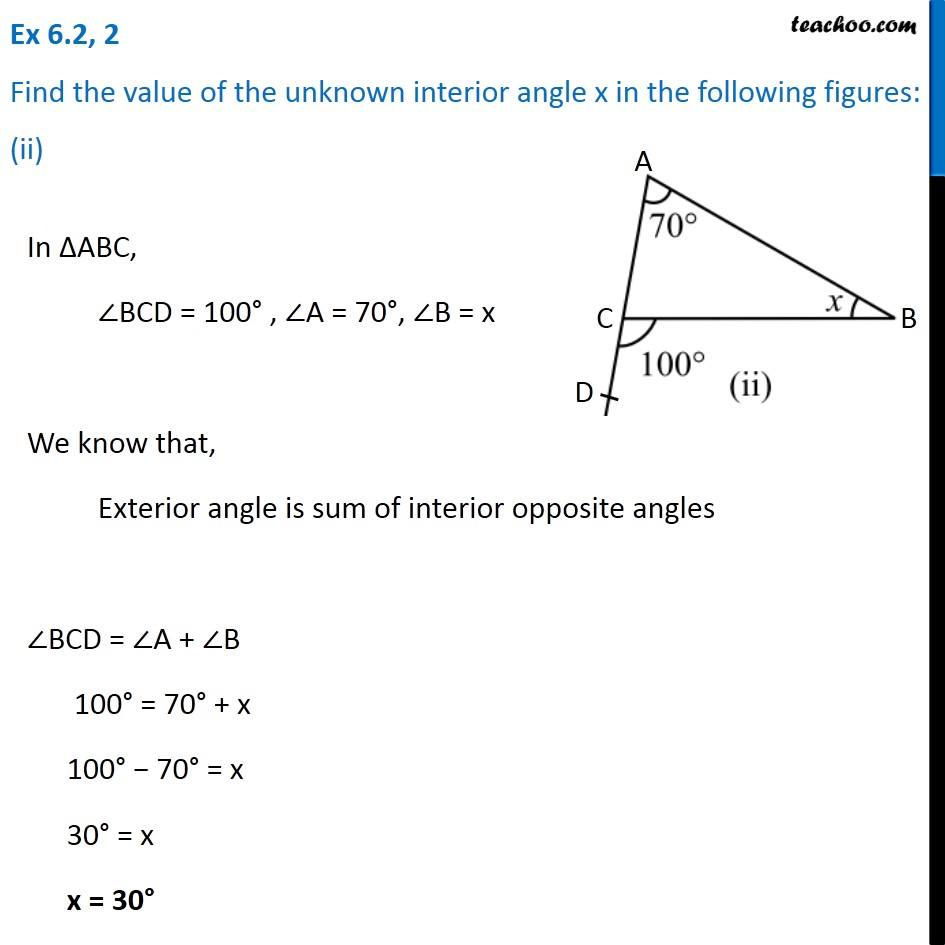Ex 6.2

Chapter 6 Class 7 Triangle and its Properties
Serial order wiseLearn in your speed, with individual attention - Teachoo Maths 1-on-1 Class

### Transcript

Ex 6.2, 2 Find the value of the unknown interior angle x in the following figures: (ii)In ∆ABC, ∠BCD = 100° , ∠A = 70°, ∠B = x We know that, Exterior angle is sum of interior opposite angles ∠BCD = ∠A + ∠B 100° = 70° + x 100° − 70° = x 30° = x x = 30°